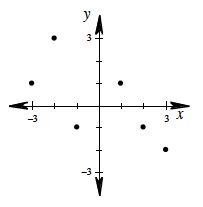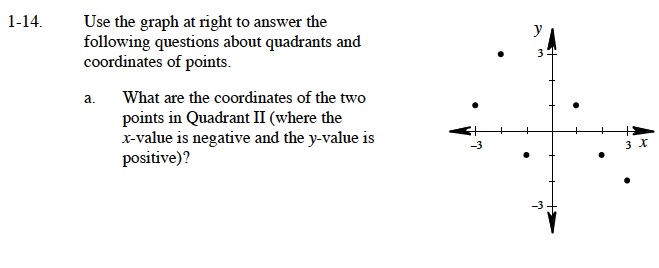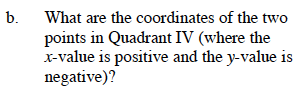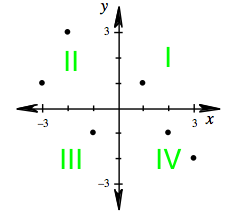### Home > CAAC > Chapter 1 > Lesson 1.1.2 > Problem1-14

1-14.
1. Use the graph below to answer the following questions about quadrants and coordinates of points. Homework Help ✎1. What are the coordinates of the two points in Quadrant II (where the x-value is negative and the y-value is positive)?

2. What are the coordinates of the two points in Quadrant IV (where the x-value is positive and the y-value is negative)?